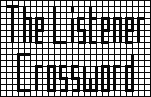## Listener Crossword 4062: Solution NotesBack to 2009 menu

## Letter Squares by Elap

### Puzzle explanation

One approach was: in 23ac K had to be 4 because any higher square would mean that 23ac had more than two digits. From 18ac U had to be 9, 16, 25 or 36, and from 2ac only U = 36 was large enough to give a four-digit value. From the clues to 18dn and 17ac the values of B and W could be fixed. After further deductions, A = 5184, B = 49, C = 1225, D = 784, E = 9, F = 3249, G = 2209, H = 412164, I = 121, J = 16129, K = 4, L = 71289, M = 64, N = 1849, O = 484, P = 2025, R = 729, S = 25, T = 225, U = 36, V = 238144, W = 324, X = 256, Y = 16 and Z = 13924. Sorting letters by ascending values gave KEYSUBMITXWORDCNPGFAZJLVH indicating that a key had to be found and that a crossword had to be submitted. Each number in the grid had to be replaced by the letter in that position in the above string so that, eg, each 14 had to be replaced by D, each 13 by R, etc.

One possible route is shown in full HERE. You will need to use the “BACK” button on your browser to return to this page.

Back to 2009 menu